# Mountain railway

Height difference between points A, B of railway line is 38.5 meters, their horizontal distance is 3.5 km. Determine average climb in permille up the track.

x =  11

### Step-by-step explanation: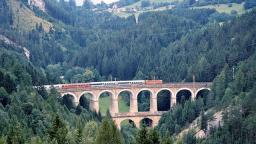Did you find an error or inaccuracy? Feel free to write us. Thank you!Tips to related online calculators
Do you want to convert length units?

#### You need to know the following knowledge to solve this word math problem:

We encourage you to watch this tutorial video on this math problem:

## Related math problems and questions:

• Railway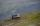Between points A, B, whose horizontal distance is 1.5 km railway line has 8promile climb. Between points B, C with horizontal distance of 900 m is climb 14promile. Calculate differences of altitudes between points A and C.
• Climb in percentageThe height difference between points A and B is 475 m. Calculate the percentage of route climbing if the horizontal distance places A, B is 7.4 km.
• RailwayRailway line had on 5.8 km segment climb 9 permille. How many meters track ascent?
• RailwaysRailways climb 7.4 ‰. Calculate the height difference between two points on the railway distant 3539 meters.
• ClimbFor horizontal distance 4.2 km road rise by 6.3 m. Calculate the road pitch in ‰ (permille, parts per thousand).
• Two municipalities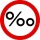The horizontal distance between municipalities is 39 km. Average sinking is 7 per mille. What is the difference in height between these municipalities?
• The railway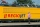The railway line has a gradient of 12 per mille. How many meters will it ascend to a horizontal distance of 4 km?Between cities A and B is route 13 km long of stúpanie average 7‰. Calculate the height difference of cities A and B.
• Slope of trackCalculate the average slope (in permille and even in degrees) of the rail tracks between Prievidza (309 m AMSL) and Nitrianske Pravno (354 m AMSL), if the track is 11 km long.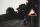A 5 km long road begins at an altitude 500 meters above sea level and ends at an altitude of 521 ASL. How many permille road rises?
• Altitude difference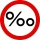What a climb in per mille of the hill long 4 km and the altitude difference is 6 meters?
• AMSL and skiingTomas skis from point A (3200m above sea level to place B. Hill has 20% descent. The horizontal distance between the start and finish is 2,5km. At what altitude is point B?
• Cablecar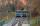Funicular on Petrin (Prague) was 408 meters long and overcomes the difference 106 meters in altitude. Calculate the angle of climb.
• ClimbOn the road sign, which informs the climb is 8.7%. The car drive 5 km along this road. What is the height difference that the car went to?
• LiftThe largest angle at which the lift rises is 16°31'. Give climb angle in permille.
• Trains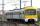On a double-track line between stations, K and M went against each other two trains. The first train passed the distance between stations for 3.5 hours, the second, which had an average speed of 12 km/h more, passed for 3.05 hours. Calculate the distance
• Height differenceWhat height difference is overcome if we pass a road 1 km long with a pitch21 per mille?